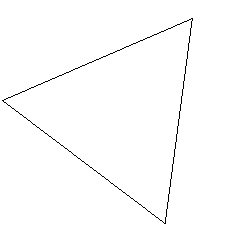﻿ The Gauss – Bonnet Theorem
Back to Math 226B page

The Gauss – Bonnet Theorem

This course is about a profound and far reaching generalization of the Gauss-Bonnet Theorem, or perhaps given how far-reaching the generalization is, it might better be said that the Gauss-Bonnet Theorem is the simplest non-trivial instance of the result (the Atiyah-Singer Index Theorem) we are aiming at.  The proof of the G-B Theorem that generalizes this far is quite different from any of the usual proofs of G-B.  But still, the G-B Theorem is the acorn from which the oak grows.  So it seems reasonable to state and prove it first by more usual, easier proofs before we begin the large scaled general program.

The Gauss Bonnet Theorem:  If M is a compact surface with a Riemannian metric, then

Where K =Gauss curvature ,  = the Euler characteristic of M and dA=the area measure on  determined by the Riemannian metric.

The Gauss-Bonnet Theorem is an “intrinsic” theorem. Whether or not the compact surface admits an isometric (or even just differentiable embedding)  in  plays no role in the statement.  But the Gauss-Bonnet Theorem is particularly easy to prove in case the surface is isometrically embedded in , so we discuss this first:

If  is a (necessarily orientable) compact embedded surface in , then one can define the “Gauss map” , by choosing a  unit normal field N on M and setting .  It is an immediate consequence of the  formula that we established last term that  .

Now from this

If we can show that   , we obtain the Gauss-Bonnet formula immediately.  To check this, let  be such that both  and  are regular values of  .

[Choice possible by Sard’s Theorem: (critical values  -(critical values)) has measure 0.]

Then  has critical points exactly at points x with  .  Moreover, the index of such a critical point is 0 or 2 at points  where  is orientation-preserving and 1 where  is orentation-reversing.

( This is just an observation using the  “normal form” of surfaces graphed over their tangent plane).

Thus,  =

where the last equality is standard Morse/CW complex theory!

So the Gauss-Bonnet follows in this case.

The general case requires a different procedure.  Suppose T is a geodesic triangle contained in a simply connected neighborhood which eventually we shall take to be small relative to something along the lines of injectivity radius.  Then we shall prove the result that

(sum of the interior angles of T) -

This fact is actually familiar in the case of  .     and area of a spherical triangle = sum of interior angles - .[The proof of this is simple :  find the area of a “lune”—the area bounded by two great circles in terms of its angle  to be , then look at the 2x three lunes determined the edges of the triangle T. Note that  (all lunes’ areas) = 2x2 (sum of interior angles) while the  all lunes =sphere with T and its antipodal image covered twice.

So, 4(area of T) + 4  = 4(sum of interior angles ) or area of T=sum of interior angles - . ]

Now suppose we have a triangulation with each triangle satisfying this formula.  Let f=number of triangles, e=number of edges, v=number of vertices.  Since each edge lies in two faces while each triangle face has three edges,

Also the sum of all the interior angles of all the triangles =  since the sum at each vertex =  and each angle occurs at a unique vertex.  Thus,

Thus the Gauss-Bonnet Theorem is established once we have proved the  formula for “small” triangles and have shown that every compact surface has a triangulation by such triangles.

The calculation required is easiest in a special kind of coordinate system.

Let  be an arc length parameter geodesic and N(t) be a unit parallel field along  with .  The function  is a diffeomorphism in a neighborhood of (0,0) onto a neighborhood of , by the Inverse Function Theorem .

In these coordinates, the u-constant, v varies curves are geodesics.  Hence

and

while  .   So  .

Write   .

Now  since  is unit  to  and the v-curves are geodesics (or calculate directly).  Thus we can calculate as follows:

Let  = angle from  to  along an arc-length parameter geodesic .  Then  and

So  where

since    so .

Now  while

So  and  so

.  Then  .

Then   .Now integrate  around a geodesic triangle T.

+sum of exterior angles of T

=total turning relative to

(easy homotopy argement) [Note that we are measuring angle C clockwise from   !]

Thus

Or

Or

.Finally, we need to establish that a suitable triangulation exists.  For this take a sufficiently dense finite set  , let  set of pts such that the distance to  <distance to any other p.  Then S is bounded by a geodesic polygon, star shaped relative to  from which triangulation follows: just connect  to the vertices of the boundary polygon of S.